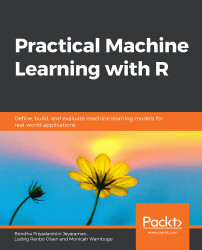•#### Practical Machine Learning with R#### Overview of this book

With huge amounts of data being generated every moment, businesses need applications that apply complex mathematical calculations to data repeatedly and at speed. With machine learning techniques and R, you can easily develop these kinds of applications in an efficient way. Practical Machine Learning with R begins by helping you grasp the basics of machine learning methods, while also highlighting how and why they work. You will understand how to get these algorithms to work in practice, rather than focusing on mathematical derivations. As you progress from one chapter to another, you will gain hands-on experience of building a machine learning solution in R. Next, using R packages such as rpart, random forest, and multiple imputation by chained equations (MICE), you will learn to implement algorithms including neural net classifier, decision trees, and linear and non-linear regression. As you progress through the book, you’ll delve into various machine learning techniques for both supervised and unsupervised learning approaches. In addition to this, you’ll gain insights into partitioning the datasets and mechanisms to evaluate the results from each model and be able to compare them. By the end of this book, you will have gained expertise in solving your business problems, starting by forming a good problem statement, selecting the most appropriate model to solve your problem, and then ensuring that you do not overtrain it.Free Chapter
An Introduction to Machine LearningData Cleaning and Pre-processingFeature EngineeringIntroduction to neuralnet and Evaluation MethodsLinear and Logistic Regression ModelsUnsupervised LearningAppendix## Introduction

While neural networks are often better than linear and logistic regression models at solving regression and classification tasks, respectively, they can be very difficult to interpret. If we wish to test the hypothesis that people drink more water when the temperature rises, it's important that we can extract this information from our model. A neural network with many layers might be very good at predicting the water consumption of a person, based on features such as age, gender, weight, height, humidity, and temperature, but it would be difficult to say how temperature alone affects the prediction. Linear regression would tell us specifically how temperature contributed to the prediction. So, while we might get a worse prediction, we gain an insight into the data and, potentially, the real world. Logistic regression, which we use for binary classification, is similarly easier to interpret.

In this chapter, we will implement and interpret linear and logistic regression models...